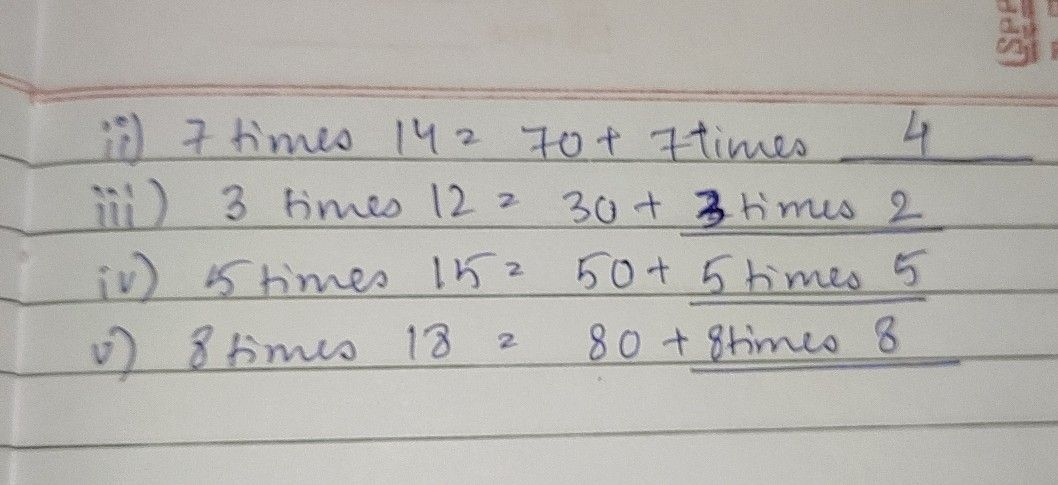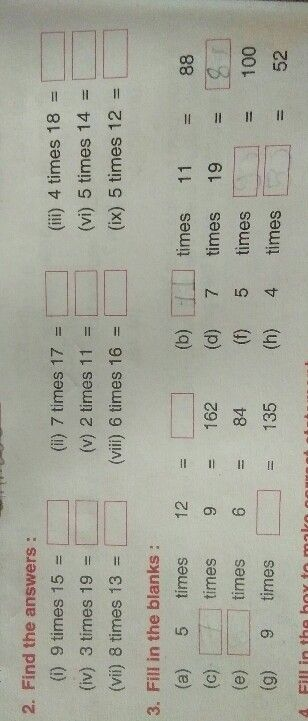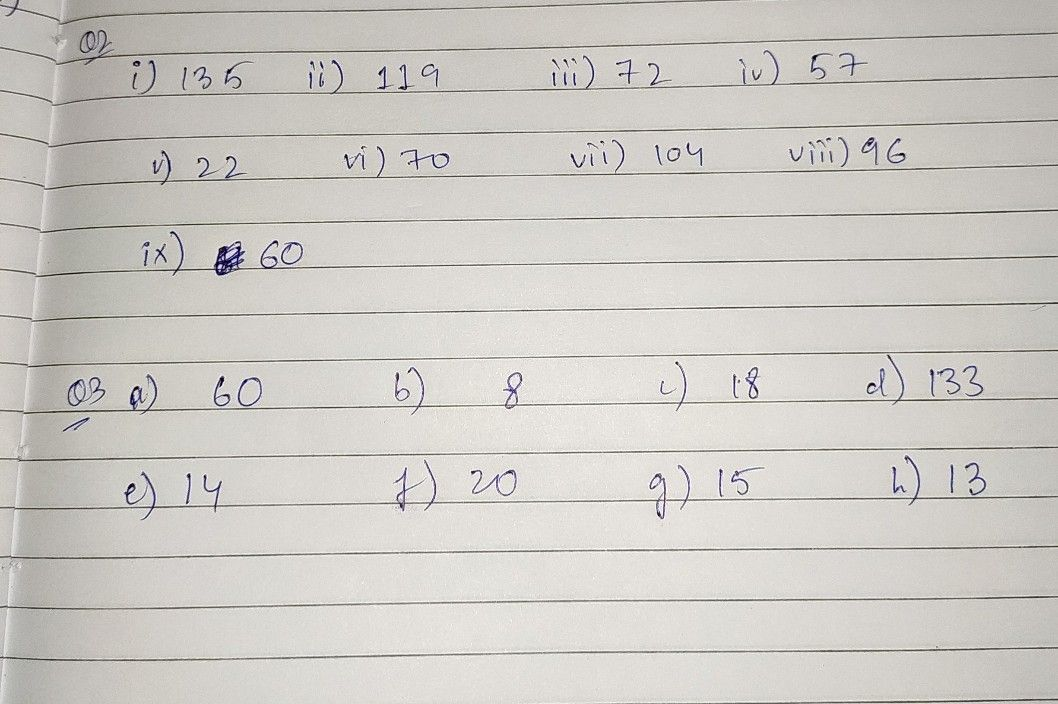Symbol
Problem6 times $16=6$ times $10+6$ times $\vec{p}$ times $14=70+7$ times 3times $12=30+$ $5$ 5 times $15=50+$ 0 $8$ 8 times $18=80+$
10th-13th grade
Other
Question content
6times 16 = 6times 10+6times
SolutionQanda teacher - gaurav sirhere is your answer
i hope it will help you
please rate and reviewStudentanswer meQanda teacher - gaurav sir
i am allowed to answer
only one question per bid